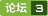### 求空间直线上点坐标 [问题点数：100分]def point(x0,y0,x1,y1,x2,y2,x3,y3): n a = y1-y0 n b = x1*y0-x0*y1 n c = x1-x0 n d = y3-y2 n e = x3*y2-x2*y3 n f = x3-x2 n y = float(a*e-b*d)/(a*f-c*d) n x = float(y*c-b)/a

<em>直线</em>表达假设<em>直线</em>都用方向和给定点表示： lil_i 的方向为 Wi=(wi1,wi2,wi3)TW^i=(w_1^i,w_2^i,w_3^i)^T, 经过点 Xi=(ai,bi,ci)TX_i=(a_i,b_i,c_i)^T, 且 ∑j(wij)2=1\displaystyle\sum\limits_j{\left(w_j^i\right)^2}=1。求两个异面<em>直线</em>之间公垂线段的中点。几何意义这个点

Intersection程序说明 该程序是应聘同豪土木工程咨询公司时出的一个小题目。 该程序使用Fortran编制，用于判断由<em>空间</em>四点表示的两<em>直线</em>是否相交,若相交则求出交点. 输入说明: 输入文件名为input.txt; 其中数据分别为:确定<em>直线</em>1所需两点<em>坐标</em>(x1,y1,z1),(x2,y2,z2); 确定<em>直线</em>2所需两点<em>坐标</em>(x3,y3,z3),(x4,y4,z4). 输出说明: 分别输出至屏幕及文件; 输出文件名为output.txt; 输出读入的四点<em>坐标</em>,以检查数据; 输出两<em>直线</em>向量及参数方程; 输出两<em>直线</em>的<em>空间</em>相对位置(共面或异面); 若共面,输出两<em>直线</em>的平面相对位置(重合、平行或相交); 若相交,输出交点<em>坐标</em>. 步骤: 1.判断是否共面 2.若共面，判断平行、重合还是相交 3.若相交，求交点

matlab 求点到任意直线的投影点坐标
【写在前面】因为图像<em>坐标</em>系和我们平时用的直角<em>坐标</em>系还是有不同的。因此在求点到<em>直线</em>投影点<em>坐标</em>的时候，不敢随便把<em>直线</em>用点斜式表示。为此采用向量的方法求投影点。公式推导代码实现function proj_point = ProjPoint( point,line_p )nx1 = line_p(1);ny1 = line_p(2);nx2 = line_p(3);ny2 = line_p(4);x3 =

emmm这个我今天百度了好久，发现都是同一篇，而且很长懒得看。。。于是我自己想了一个nn设四个点构成两个线段p1(x1,y1,z1) p2(x2,y2,z2) p3(x3,y3,z3) p4(x4,y4,z4) ，向量a=p2-p1,b=p4-p3; n1.先判断两个线段是否平行 n2.再计算d = cross(a,b); //就是同时与两<em>直线</em>垂直的向量 n3.根据 p1和d 确定一个平面，计算...

C++球两<em>直线</em>的交点MyPoint setpoint(Line &l,Line &m) { MyPoint p; p.x=(m.b-l.b)/(l.a-m.a); p.y=(l.a*m.b-m.a*l.b)/(l.a-m.a); return p; } 这的MyPoint p; 要加两个参数 而且不能直接用p.x p.y

inline cVector3d cProjectPointOnLine(const cVector3d& a_point,n const cVector3d& a_pointOnLine,n const cVector3d& a_directionOfL

//<em>直线</em>过P1,P2两点，P0为<em>直线</em>外一点，求P0到<em>直线</em>的距离和P0在<em>直线</em>上的垂足void HelloWorld::initPoint(cocos2d::CCPoint point0,cocos2d::CCPoint point1,cocos2d::CCPoint point2){ P1 = point1; //<em>直线</em>上的第一个点 P2 = point2; //<em>直线</em>上的第二个点 P0 = point

-
C++实现三维空间中点到点、点到直线、点到平面的距离计算
C++实现三维<em>空间</em>中点到点、点到<em>直线</em>、点到平面的距离计算。

python 求直线交点坐标
python 求<em>直线</em>交点<em>坐标</em>

【寒江雪】计算直线与平面的交点坐标

c++求两条线段的交点坐标
c++求一个平面内，两条线段的交点<em>坐标</em>。传入四个<em>坐标</em>点，返回一个<em>坐标</em>点。

hough 直线提取

//获取一个点到另外两个点所在<em>直线</em>的投影。 如果点在<em>直线</em>上，返回其本身 //下面公式适用于二维<em>空间</em>的点， Y轴的数值不考虑public static Vector3 GetProjectToLine(Vector3 _Point, Vector3 L0Point, Vector3 L1Point)n {n //去掉Y轴n _Point.y = 0;n

C++语言实现一些基本算法(两点距离、点是否在直线上、点与直线的关系、两直线的夹角、两直线的交点、两个举行的重合面积等等)
C++语言实现一些基本算法(两点距离、点是否在<em>直线</em>上、点与<em>直线</em>的关系、两<em>直线</em>的夹角、两<em>直线</em>的交点、两个举行的重合面积等等)

1、已知<em>直线</em>上两点求<em>直线</em>的一般式方程nn已知<em>直线</em>上的两点P1(X1,Y1) P2(X2,Y2)， P1 P2两点不重合。则<em>直线</em>的一般式方程AX+BY+C=0中，A B C分别等于：nnA = Y2 - Y1nnB = X1 - X2nnC = X2*Y1 - X1*Y2nn2、过<em>直线</em>外一点P0(x0,y0）的垂线方程：y=(B/A)*(x-x0)+y0nn3、求<em>直线</em>与垂线的交点nnx=((B^2)...
pcl::lineToLineSegment() 计算空间直线的交点和最小公垂线
对于两<em>空间</em><em>直线</em>来说，计算交点和最小公垂线是一码事，交点即最小公垂线两个垂足的中心。PCL中源码中包含计算<em>空间</em><em>直线</em>最小公垂线的函数nnnnPCL_EXPORTS void pcl::lineToLineSegment (const Eigen::VectorXf &amp;amp; line_a,n cons...
C语言编写程序计算圆上的点的坐标
Problem DescriptionnThere is a cycle with its center on the origin.nNow give you a point on the cycle, you are to find out the other two points on it, to maximize the sum of the distance between each ...

1、线段与线段求交点n已知线段的起点和终点，求交点，这个比较简单，解2个二元一次方程可以求出。na、我这里的算法是判断2条线段的定义域和值域是否有重合地方，有则进行下一步判断，没有这返回空，表示没有交点。nb、根据<em>直线</em>方程2点式y - y1 = (y2 - y1) / (x2 - x1)(x - x1)，进行解方程，不过这之前先进行对斜率判段，分为斜率k不存在，k=0，k存在三种情况，n当

2018-01-17 创建人：Ruo_Xiaon邮箱：xclsoftware@163.comnn1、一般方程：两个相交的平面确定一条<em>直线</em>。nnnn2、点向式：点和<em>直线</em>方向可以确定一条<em>直线</em>。nnnn3、两点式：<em>空间</em>两个点确定一条<em>直线</em>。

【CV】3D空间中椭球曲面与直线的交点问题

<em>直线</em>方程 y=kx+b;n圆标准方程 (x-a)2+(y-b)2=r2npublic class PointVn{n public double x { get; set; }n public double y { get; set; }n}nn /// &amp;lt;summary&amp;gt;n/// 计算与圆相交<em>坐标</em>n/// &amp;lt;/summary&amp;gt;n/// &amp;lt;para...

Point2d  pointOfIntersection(Point2d l1, Point2d l11,Point2d l2, Point2d l22)rn{rnPoint2d lastP;rnrndouble k1,k2,b1,b2;rnrnrn//两条非垂<em>直线</em>rnif(l1.x-l11.x!=0 && l2.x-l22.x!=0)rn{rnb1=(l1.y*l11.x-l11.y*l1.x)

C++实现三维空间直线间距离的计算
C++实现三维<em>空间</em>中<em>直线</em>间距离的计算n三维欧几里得<em>空间</em>中，<em>直线</em>的关系为：相交、平行和异面<em>直线</em>三种情况。本文采用向量方程来定义<em>直线</em>，向量的叉乘、点乘来计算<em>直线</em>间距离。程序用C++实现。
JS：求点与线段的最短距离，并返回该最短距离在线段上的坐标

rn rn 求圆和<em>直线</em>之间的交点rn rn rn rn n/**n * 求圆和<em>直线</em>之间的交点n * <em>直线</em>方程：y = kx + bn * 圆的方程：(x - m)² + (x - n)² = r²n * x1, y1 = 线<em>坐标</em>1, x2, y2 = 线<em>坐标</em>2, m, n = 圆<em>坐标</em>, r = 半径n */npublic getInsertPointBetweenCircleAnd...

528_计算圆弧上某一点的坐标

c++计算三维空间中任意两条直线之间的位置关系（平行、相交、交错、重合）

【C\C++】空间中求一点到两点所构成的直线的距离

matlab计算缓圆曲线上各点坐标点的程序

C++根据两点式方法求直线并求两条直线的交点

C++ 实现已知直线上两个点求解直线方程，并求两条直线的交点

python 计算两直线交点

<em>空间</em><em>直线</em>标准方程：nnn转化为射影式方程：nnn可以对两个方程分别进行拟合。n令：nnnn其中nn求出a,b,c,d即可。n代码如下：ndata = load('data.txt');rndata = data';rnL=length(data(1,:));rnx=data(1,:);rny=data(2,:);rnz=data(3,:);rnF=[z;1 1 1 1 1 1 1 1 1 1 1]

1、pp为所求的投影点<em>坐标</em>；n2、A为平面上任意已知点；n3、n为平面上的法线；nn的计算方法：n一般会已知平面上两个以上的点<em>坐标</em>，例如我是为了求点在任意三角形上的投影点，我当然会n知道三角形的三个点<em>坐标</em>，通过其中两个点<em>坐标</em>可以求出法向量n。nnn假设知道三角形的三个顶点A（x,y,z）,B(x,y,z),C(x,y,z).nnAB = (Bx-Ax,By-Ay,Bz-Az);
Opencv学习笔记-----求取两条直线的交点坐标

1. 前言最近老板让写一段<em>空间</em>点匹配的代码， 其中涉及到求<em>空间</em>两<em>直线</em>之间的距离，写起来满费劲的， 这里做一个记录。2. 处理思路<em>空间</em>两<em>直线</em>之间的位置关系主要可以分为： 重合， 平行， 相交， 异面。2.1 异面情形（含相交）：n 已知<em>空间</em>中两线段，如果它们无限变粗，判断是否相交。（主要讨论不在同一平面的情况）线段AB 线段CD n 问题的关键是求出这两条任意<em>直线</em>之间的最短距离，以及在这个距离上的
AS3中求一条直线上点坐标

local point1 = {n ['x'] = -1,n ['y'] = 0,n}nnlocal point2= {n ['x'] = -1,n ['y'] = 1,n}nn-- 获取斜截式<em>直线</em>（垂直x的<em>直线</em>不适用斜截式，因为斜率无限大）nlocal GetKB = function(p1, p2)n if p1.x == p2.x then return endn local k, bn k
[算法]直线与圆的交点程序设计
/**n * Created by apple on 2017/1/15.n */n//求<em>直线</em>与圆的交点n/*函数参数说明:n cx:圆X轴<em>坐标</em>n cy:圆y轴<em>坐标</em>n r:圆半径n stx:起点<em>直线</em>的X轴<em>坐标</em>n sty:起点<em>直线</em>的轴<em>坐标</em>n edx:终点<em>直线</em>的X轴<em>坐标</em>n edy:终点<em>直线</em>的Y轴<em>坐标</em> 返回值:交点<em>坐标</em>(x,y)n*/nfunction getPoint(cx,cy,r
C# 计算两条线段交点的位置

OpenCV 遍历两点直线上的所有点

poj 1269判断两条直线的位置关系 以及求交点

【C#】点到线段最短距离的那条直线与线段的交点
/// n /// 点到线段最短距离的那条<em>直线</em>与线段的交点，{x=...,y=...}n /// n /// 线段外的点的x<em>坐标</em>n /// 线段外的点的y<em>坐标</em>n /// 线段顶点1的x<em>坐标</em>n /// 线段顶点1的y<em>坐标</em>n /// 线段顶点2的x<em>坐标</em>n /// 线段顶点2的y<em>坐标</em>n /// n public Vector2 PointFo
【寒江雪】空间内两直线相交
<em>空间</em>内两<em>直线</em>相交nn  在三维<em>空间</em>内，两<em>直线</em>相交公式的推导。整个推导过程假设两<em>直线</em>一定相交。 n n设<em>空间</em>内有<em>直线</em>l1,l2l1,l2l_1,l_2相交于点pppnl1=t1a1→+b1l1=t1a1→+b1l_1 = t_1\vec{a_1}+b_1 nl2=t2a2→+b2l2=t2a2→+b2l_2 = t_2\vec{a_2}+b_2 np=t2a2→+b2p=t2a2→+b2p = t_...

n n n 将AB写成参数式A+tv(v为向量AB)，设Q的参数为t。， 那么Q=A+vt。，由点积为0可得Dot(v,p-(A+vt。)) = 0,这样就可以解出t。nPoint getlineprojection(Point P, Point A, Point B){n Vector v = B-A;n return A+v*(Dot(v,P-A) /...

Web Api 的 路由机制
ASP.NET Web API 是一种框架，用于轻松构建可以访问多种客户端（包括浏览器和移动设备）的 HTTP 服务。 ASP.NET Web API 是一种用于在 .NET Framework 上构建 RESTful 应用程序的理想平台。所以我们要想学习web api 就要去了解它的路由机制。MVC和web api的路由机制的主要区别是web api 使用的是http 方法而不是url 的路径。本